# Equation + natural numbers - math problems

#### Number of problems found: 141

• Unknown numbersThe sum of two consecutive natural numbers and their triple is 92. Find these numbers.
• AM of three numbersThe number 2010 can be written as the sum of 3 consecutive natural numbers. Determine the arithmetic mean of these numbers.
• Two numbers 6Fill two natural numbers a, b: 7 + blank- blank = 5
• Sum of four numbers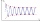The sum of four consecutive natural numbers is 114. Find them.
• Find twoFind two consecutive natural numbers whose product is 1 larger than their sum. Searched numbers expressed by a fraction whose numerator is the difference between these numbers and the denominator is their sum.
• Count of roots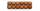How many solutions has equation x. y = 7757 with two unknowns on the set of natural numbers?
• Sum of sevenThe sum of seven consecutive odd natural numbers is 119. Determine the smallest of them.
• The sumThe sum of the squares of two immediately following natural numbers is 1201. Find these numbers.
• Two numbers 7The sum of two consecutive even numbers is 30. Find the numbers.
• Equation algebraogram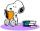Solve the equation: oco + ivo = cita How much has the task of solutions?
• Sinus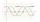Determine the smallest integer p for which the equation 4 sin x = p has no solution.
• Two identical five-digitsWe have two identical five-digit numbers, but we don't know which ones. When we write one before the first and after the second one we get two six-digit numbers, one of which is three times the other. What are the numbers?
• Diofant equationIn the set of integers (Z) solve the equation: ? Write result with integer parameter ? (parameter t = ...-2,-1,0,1,2,3... if equation has infinitely many solutions)
• Odd numbersThe sum of four consecutive odd numbers is 1048. Find those numbers ...
• Unknown number 716% of the unknown number is by 21 less than unknown number itself. Determine the natural unknown number.
• Year 2018The product of the three positive numbers is 2018. What are the numbers?
• Diofant 2Is equation ? solvable on the set of integers Z?
• Even / odd numbers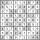a / Using variable n write two consecutive odd numbers. b / the sum of three consecutive odd numbers is 333. What are this numbers?
• Diofant equation250x + 120y = 5640
• House numbering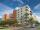The residential house has three entrances numbered even numbers, successive immediately behind. The sum of the two numbers on the outside entrances is 68. Calculate the middle of these three numbers.

Do you have an interesting mathematical word problem that you can't solve it? Submit a math problem, and we can try to solve it.

We will send a solution to your e-mail address. Solved examples are also published here. Please enter the e-mail correctly and check whether you don't have a full mailbox.

Please do not submit problems from current active competitions such as Mathematical Olympiad, correspondence seminars etc...
Do you have a linear equation or system of equations and looking for its solution? Or do you have a quadratic equation? Equations Math problems. Natural numbers - math problems.